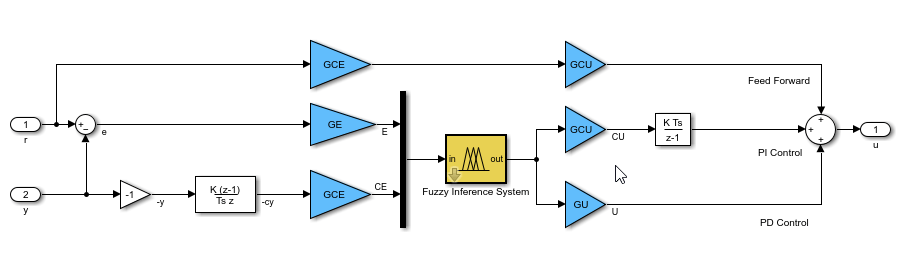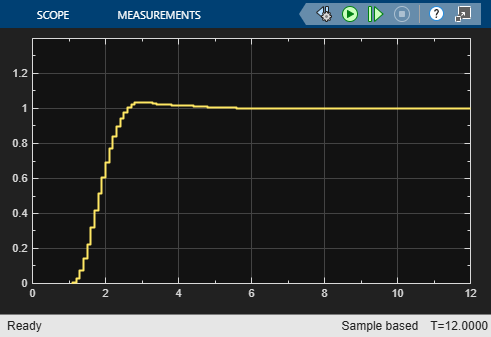Documentation

## Generate Structured Text for Fuzzy System Using Simulink PLC Coder

You can generate Structured Text for a Fuzzy Logic Controller block using Simulink® PLC Coder™. For more information on generating Structured Text, see Code Generation (Simulink PLC Coder).

While this example generates Structured Text for a type-1 Sugeno fuzzy inference system, the workflow also applies to Mamdani and type-2 fuzzy systems.

By default, the Fuzzy Logic Controller block uses double-precision data for simulation and code generation. The `fuzzyPID` model is configured to use double-precision data. You can also use either single-precision or fixed-point data. For more information on configuring your fuzzy inference system for code generation, see Fuzzy Logic Controller.

```mdl = 'fuzzyPID'; open_system(mdl) ```It is good practice to validate the performance of the system in Simulink before generating code. Run the simulation.

```sim(mdl) open_system([mdl '/Output']) ```To generate Structured Text for the model, use the `plcgeneratecode` function, which generates code for an atomic subsystem in a model. To generate code for the Fuzzy PID controller, configure the subsystem as an atomic subsystem by selecting the Treat as atomic unit parameter for the subsystem.

```subsys = [mdl '/Fuzzy PID']; set_param(subsys,'TreatAsAtomicUnit','on') ```

When generating code for just a Fuzzy Logic Controller block, place the block inside a subsystem, and set the Treat as atomic unit parameter of that subsystem.

Generate Structured Text for the Fuzzy PID subsystem.

```plcgeneratecode(subsys); ```
```PLC code generation successful for 'fuzzyPID/Fuzzy PID'. Generated files: <a href="matlab: edit('plcsrc/fuzzyPID.exp')">plcsrc/fuzzyPID.exp</a> ```

By default, the software saves the generated code in the following location:

`plcsrc/fuzzy_PID.exp`